Logarithms

Logarithms

 Site: TBAISD Moodle Course: Michigan Algebra II Preview 2012 Book: Logarithms Printed by: Guest user Date: Tuesday, September 22, 2020, 07:51 AM

Logarithms

Logarithms have many applications in the real world. Since logs can take gigantic numbers and represent them with very small numbers, they are especially useful in science. This book discusses three main applications: pH, Richter scale, and Decibels.

pH

The pH scale measures whether a solution is acidic or basic. The pH scale ranges from 0 to 14. A pH of 7 is neutral, less than 7 is acidic, and greater than 7 is basic.

The pH scale is logarithmic and as a result, each whole pH value below 7 is ten times more acidic than the next higher value. For example, a pH of 4 is ten times more acidic than a pH of 5 and 100 times (10 times 10) more acidic than a pH of 6. The same holds true for pH values above 7, each of which is ten times more basic than the next lower whole value. For example, a pH of 10 is ten times more basic than a pH of 9 and 100 times more basic than a pH of 8. pH is determined by the formula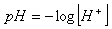Examples

Example 1 Find the pH of apple juice with a hydrogen ion concentration of 3.2 x 10-4 .

Step 1. Substitute 3.2 x 10-4 in the formula.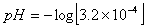Step 2. Use a calculator to solve.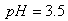Example 2
Find the hydrogen ion concentration of ketchup which has a pH of 3.9.

Step 1. Substitute 3.9 into the formula.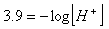Step 2. Use the inverse operations of logs and exponents and a calculator to solve.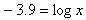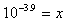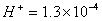Video Lesson

To learn how to calculate pH using logs, select the following link:

Calculate pH Using Logs

Interactive Activities

Explore pH

The pH Factor

The Playground below is designed to take the mystery out of pH.

Acid-Base Tutorial

Find answers to various questions about the physical structure of matter, atoms, and related subjects.

pH and Indicators

Richter Scale

The Richter scale is the best known scale for measuring the magnitude of earthquakes. The magnitude value is proportional to the logarithm of the amplitude of the strongest wave during an earthquake. A recording of 7, for example, indicates a disturbance with ground motion 10 times as large as a recording of 6. The energy released by an earthquake increases by a factor of 30 for every unit increase in the Richter scale.

When working with Richter scale problems, use the following formula: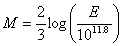where M is the magnitude and E is the amount of energy released in ergs.

Example

In 1997, a California Earthquake measured 3.2 on the Richter scale. How much energy was released?

Step 1. Substitute 3.2 into the formula for M.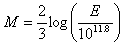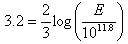Step 2. Solve the formula for E.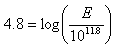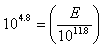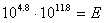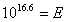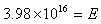The energy released by an earthquake with the magnitude of 3.2 is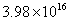ergs.

Video Lesson

To learn how to calculate Richter scale using logs, select the following link:

Calculate Richter Scale Using Logs

Decibels

The intensity of sound is measured in a unit called the decibel (dB), which describes the relative intensity of a sound based on a logarithmic scale containing values ranging from 0 to 194.

A zero value on the decibel scale represents the weakest sound audible to humans and sound intensity increases in correspondence with numeric values. However, the relationship among the values on the decibel scale is not linear but logarithmic. A sound with a 50 dB level is not twice as intense as a sound with a 25 dB level. Rather, each three decibel increment affects a 50% change in sound pressure levels. Though reducing the decibel level produced by a sound source from 80 to 77 may not seem like a major change, it would actually represent a 50% reduction in audible sound.

The intensity of a sound reaching a person's ear depends not only on the intensity of the sound produced, but also on the persons distance from the source of the sound. If you were standing one foot away from a loud machine, for instance, you would experience higher decibel levels than if you were ten feet away, even though the intensity of the sound produced remains unchanged.

To calculate decibels, use the following formula: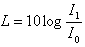, where L is loudness and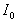is 10-12 which is barely audible.

Examples

Example 1 Find the loudness in decibels of a sound that has an intensity of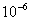.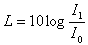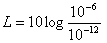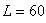dB

Example 2 Find the intensity of a sound that measures 90 dB.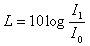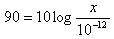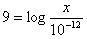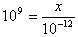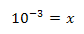Interactive Activity

How loud is too loud?

Dangerous Decibels Virtual Exhibit

Practice

Log Applications Worksheet

Sources

Sources used in this book:

Blamire, John. "pH and indicators." http://www.brooklyn.cuny.edu/bc/ahp/ChemInvest/CI.Indicators.html (accessed 7/14/2010).

Embracing Mathematics, Assessment & Technology in High Schools; a Michigan Mathematics & Science Partnership Grant Project

Grogono, Alan. "The Acid-Base Tutorial." http://www.acid-base.com/ph.php (accessed 7/14/2010).

Holt, Rinehart & Winston, "Environmental Applications." http://my.hrw.com/math06_07/nsmedia/lesson_videos/alg2/player.html?contentSrc=7145/7145.xml (accessed 7/14/2010).

Holt, Rinehart & Winston, "Geology Applications." http://my.hrw.com/math06_07/nsmedia/lesson_videos/alg2/player.html?contentSrc=7150/7150.xml (accessed 7/14/2010).

OMSI, "Dangerous Decibels." http://www.dangerousdecibels.org/virtualexhibit/index.html (accessed 7/14/2010).

Rustad, Mark. "Measuring the Intensity of Sound, Decibels Explained." http://www.articlesbase.com/science-articles/measuring-the-intensity-of-sound-decibels-explained-252817.html (accessed 7/14/2010).

"Scales for Measuring Earthquakes." http://www.matter.org.uk/schools/content/seismology/richterscale.html (accessed 7/14/2010).

"The pH Factor." http://www.miamisci.org/ph/phmilk.html (accessed 7/14/2010).